Signup

# How to add and change variables

You can use variables within Branching & calculations to add up scores or prices. You can also create your own custom variables in Number or Text format.

1. Go to the Logic tab on the right-hand side of the Create panel, open Personalize with data, then select Variables: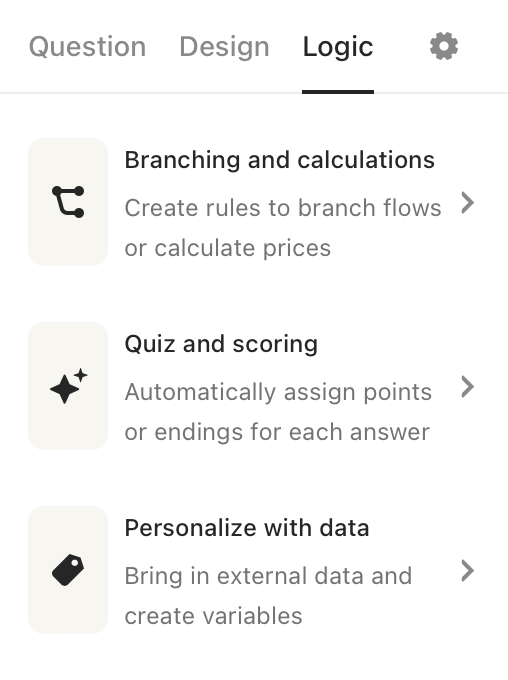2. Now you’ll see the default variables. Click + Add custom variable to create your own. Choose Number or Text from the drop-down menu, and optionally add a base value. Hit Save when ready.

Note! Only lowercase letters, numbers, and underscores are accepted, when creating a custom variable.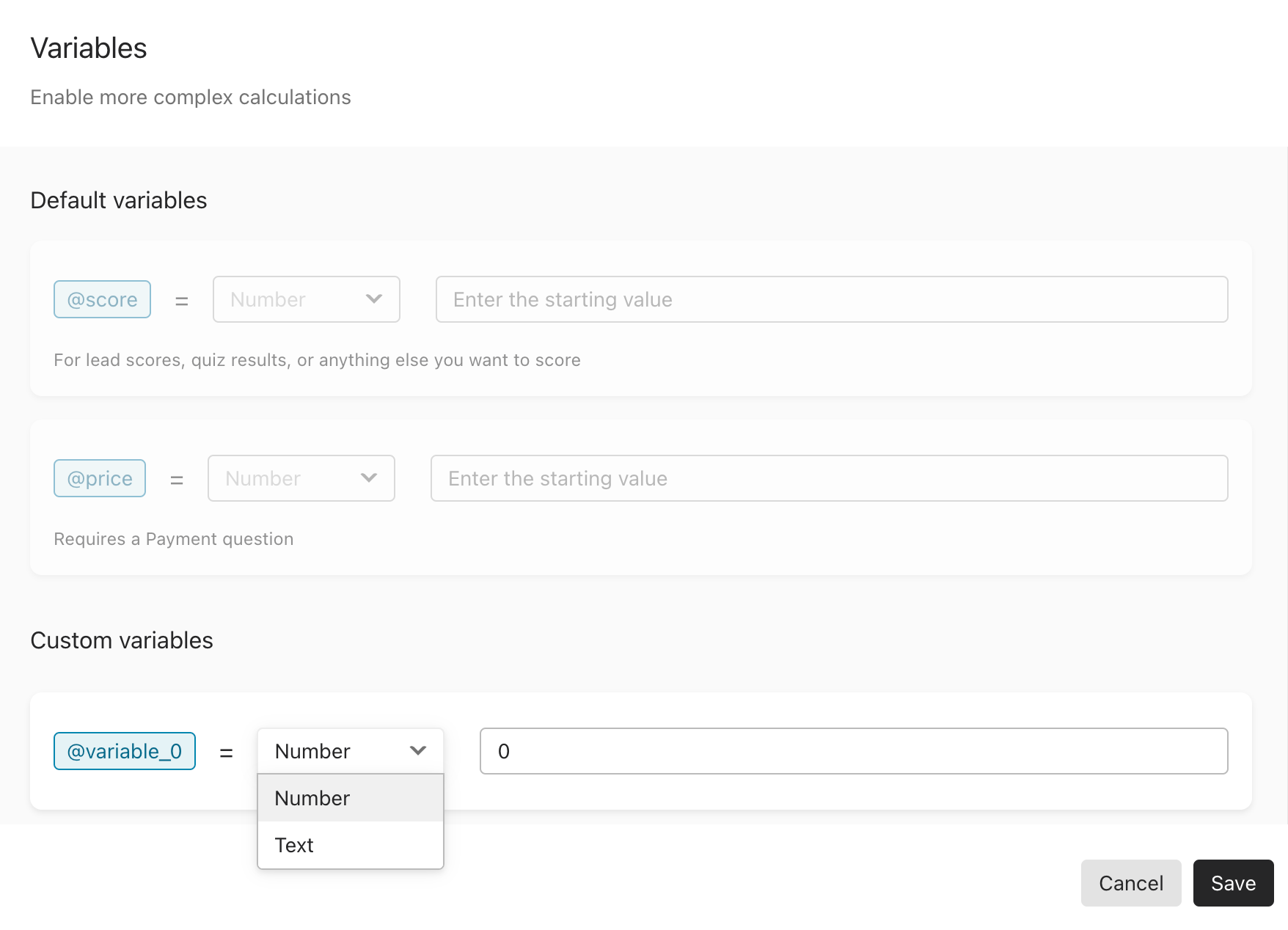######Tip: Adding a base value can be useful for defining a unit price or a default text string that you’d like to display in your typeform later on.

You’ll always see the price and score Variables in the list, but they are greyed out by default. The price Variable will become available when you add a Payment question to your typeform, and the score Variable will become available when you add scores.

You can show a variable’s current value in your typeform questions by typing @ and using Recall information.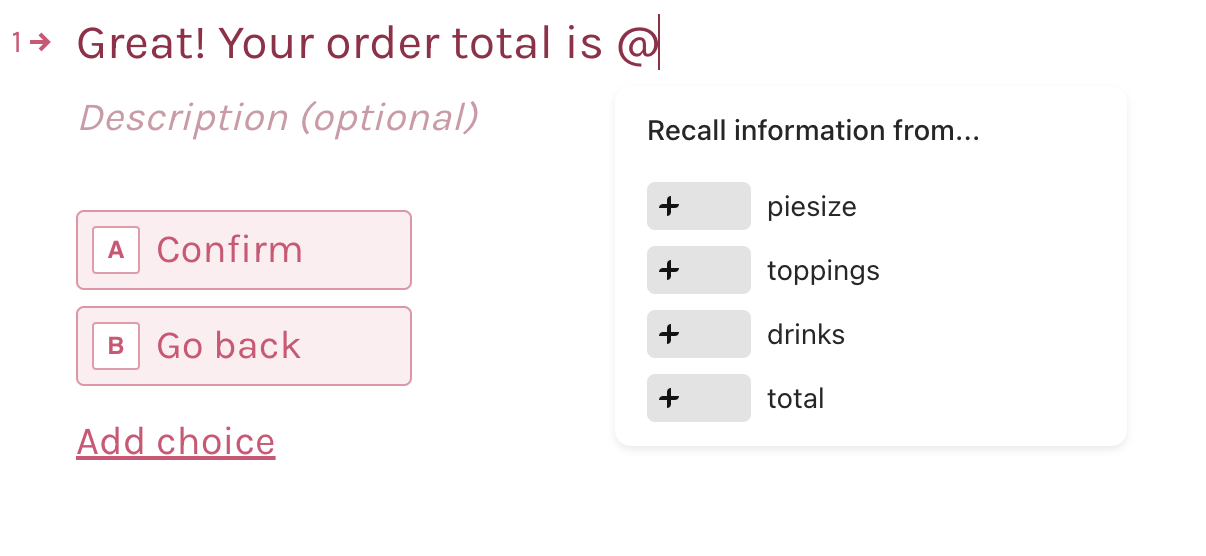### Changing the value of Number variables

You can change the value of Number variables by adding rules in the Branching and calculations menu, in the Logic tab.1. Open the branching menu and click + Add rule by the question you want to edit.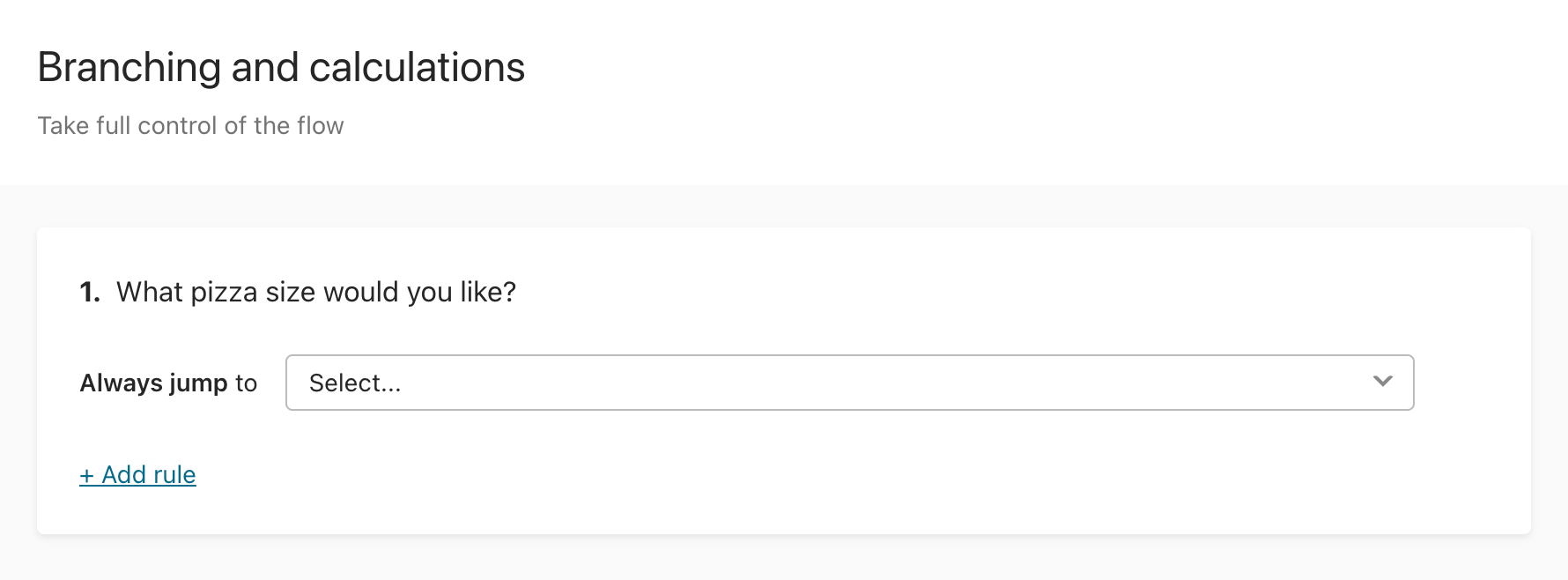2. Switch from “if” to “always” in the first dropdown menu to always change the variable’s value when that question is answered.

In the next dropdown menus, choose a math operation to apply to the variable, the variable you’d like to change, and the factor you’d like to change it by. This factor could be another variable, the question answer, or a number you choose.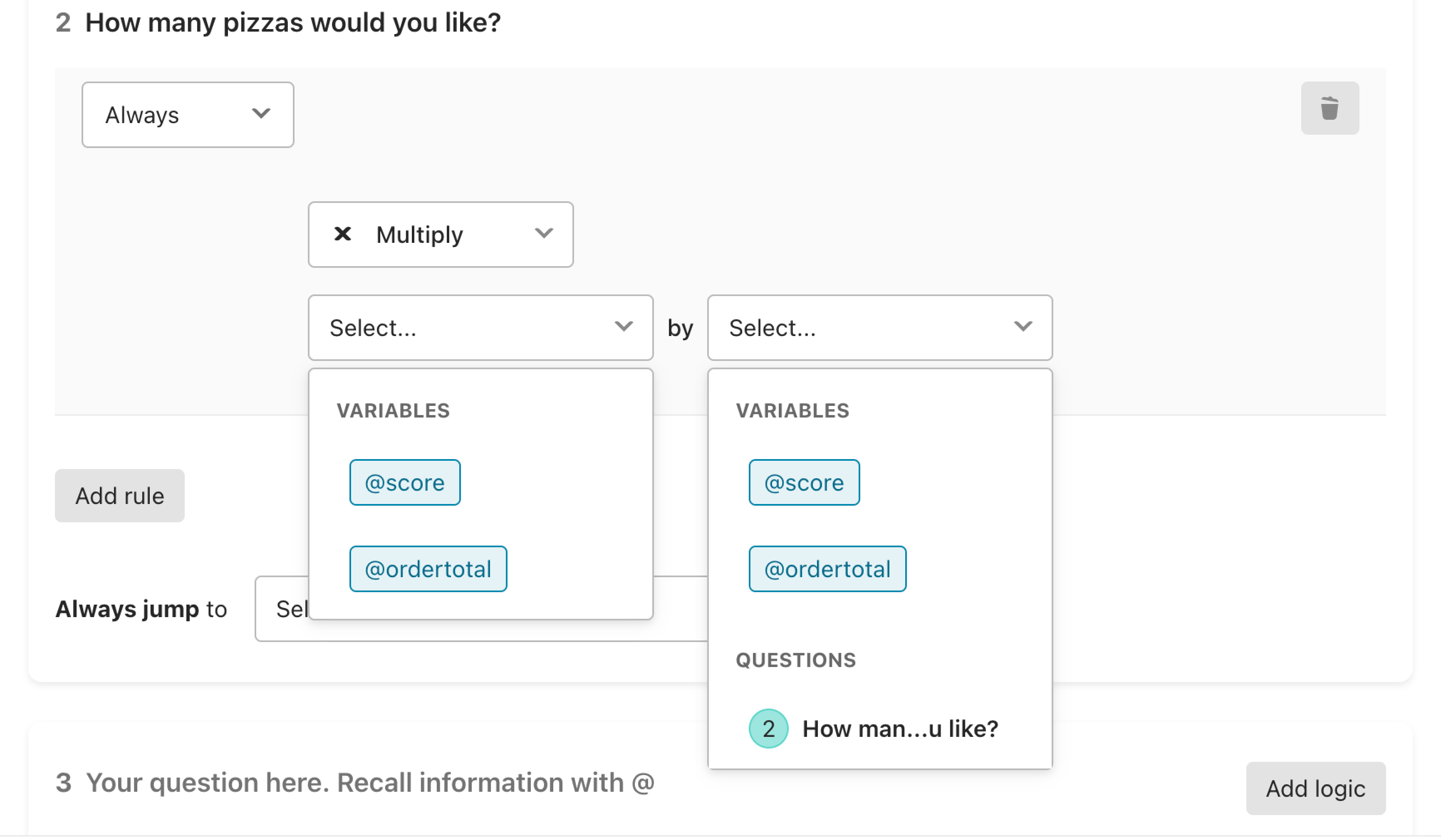For example, you might create a rule like this for a question like “How many pizzas would you like?” You can then multiply an order price variable by the respondent’s answer: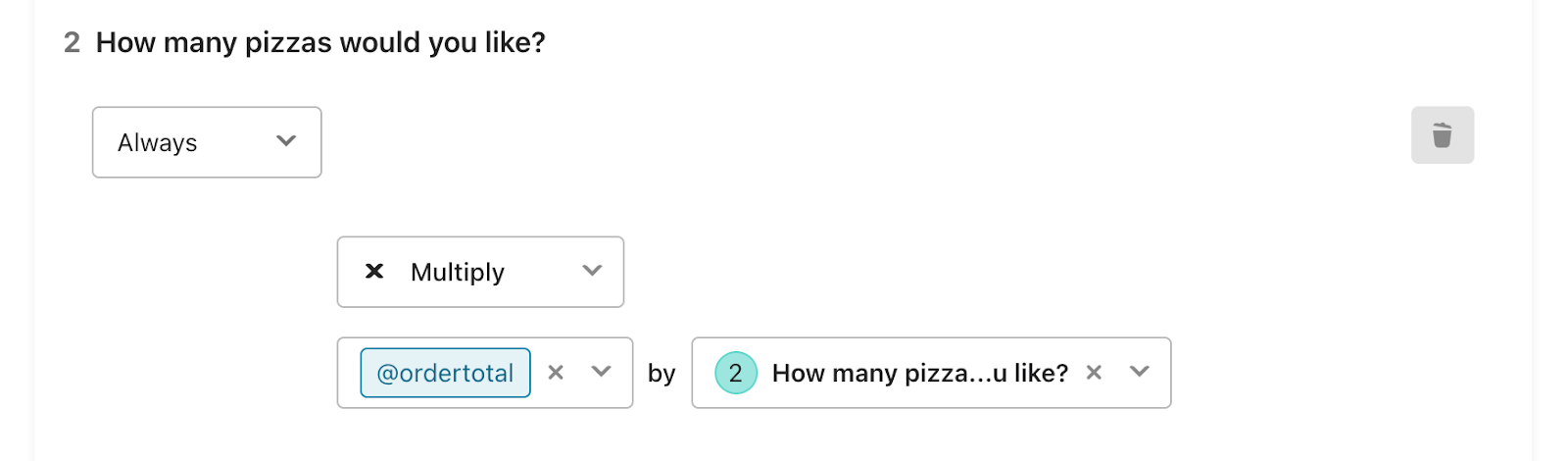3. To only change the variable’s value in certain cases, choose “if” in the first dropdown menu. Add the appropriate condition before setting up the rest of the rule.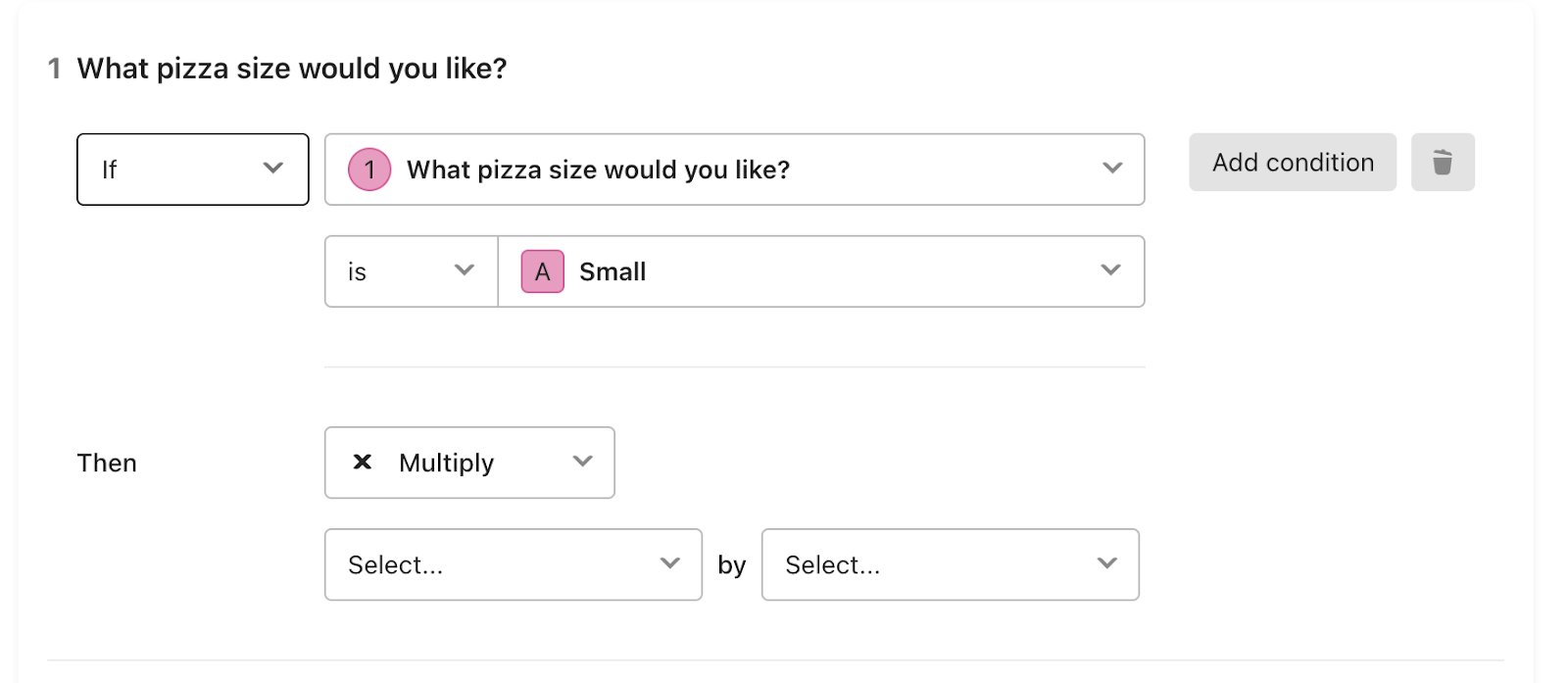For example, you can create a rule like this for the question “What pizza size would you like?” The order total variable would increase by different amounts depending on the chosen size.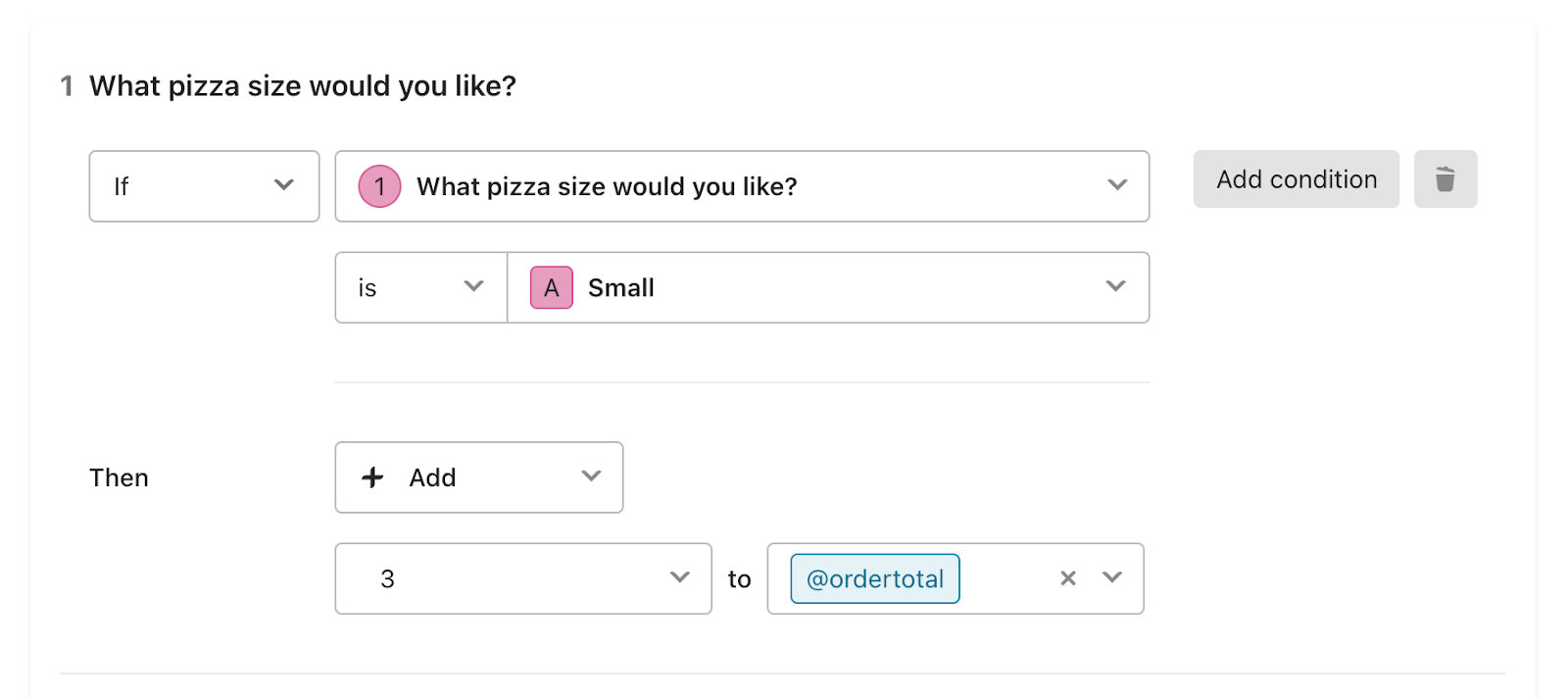4. You can use variables to change the value of other variables. For example, if you had a variable called blue and another variable called red, you could add, subtract, multiply, or divide between the two: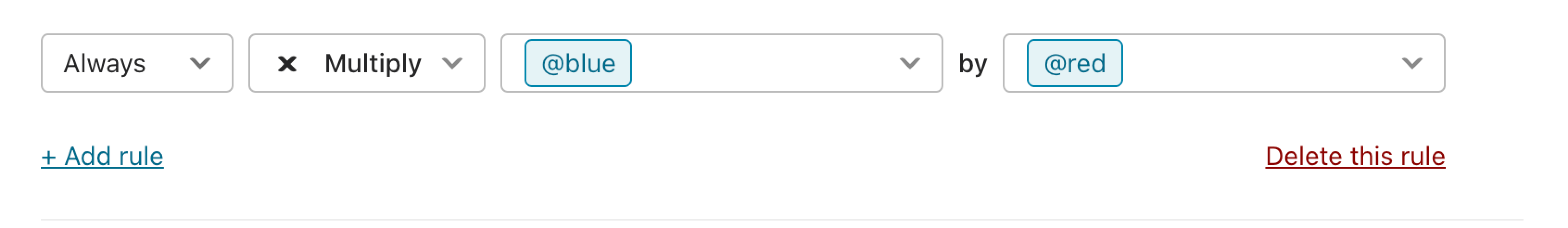How do these operations affect each variable? Let's say that you start with two variables, red, with a value of 10, and blue, with a value of 5.

• If you add blue to red, the value of red becomes 15, and the value of blue stays at 5.
• If you add red to blue, the value of blue becomes 15, and the value of red stays at 10.
• If you subtract blue from red, the value of red becomes 5, and the value of blue stays at 5.
• If you subtract red from blue, the value of blue becomes -5, and the value of red stays at 10.
• If you multiply red by blue, the value of red becomes 50, and the value of blue stays at 5.
• If you multiply blue by red, the value of blue becomes 50, and the value of red stays at 10.
• If you divide red by blue, the value of red becomes 2, and the value of blue stays at 5.
• If you divide blue by red, the value of blue becomes 0.5, and the value of red stays at 10.

These values will then apply throughout the rest of the form, unless you change them with another operation.

### Changing the value of a Text variable

You can change the value of Text variables by adding rules in the Branching and calculations menu, in the Logic tab.

1. Open the branching menu and click + Add rule by the question you want to edit.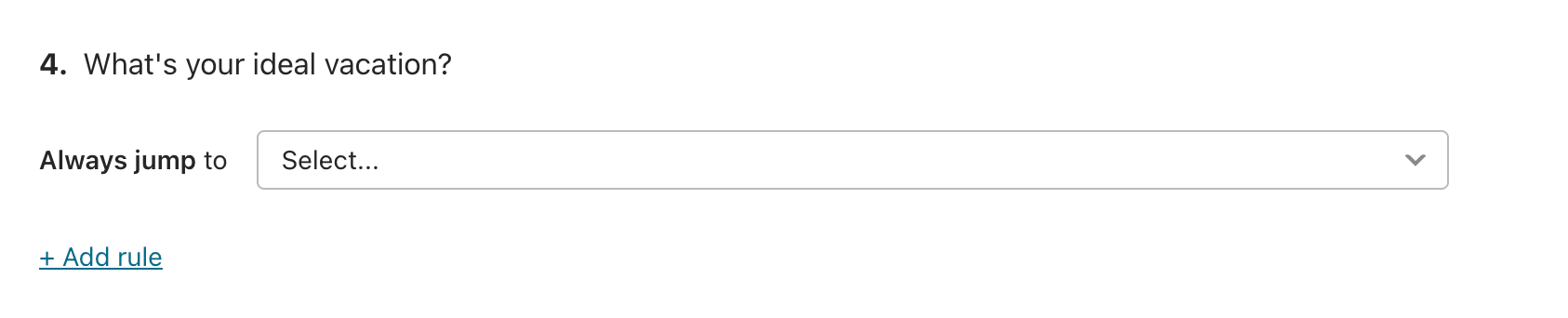2. Switch from “if” to “always” in the first dropdown menu to always change the variable’s value when the question is answered.

To change its value in certain cases only, choose “if” and add the appropriate condition.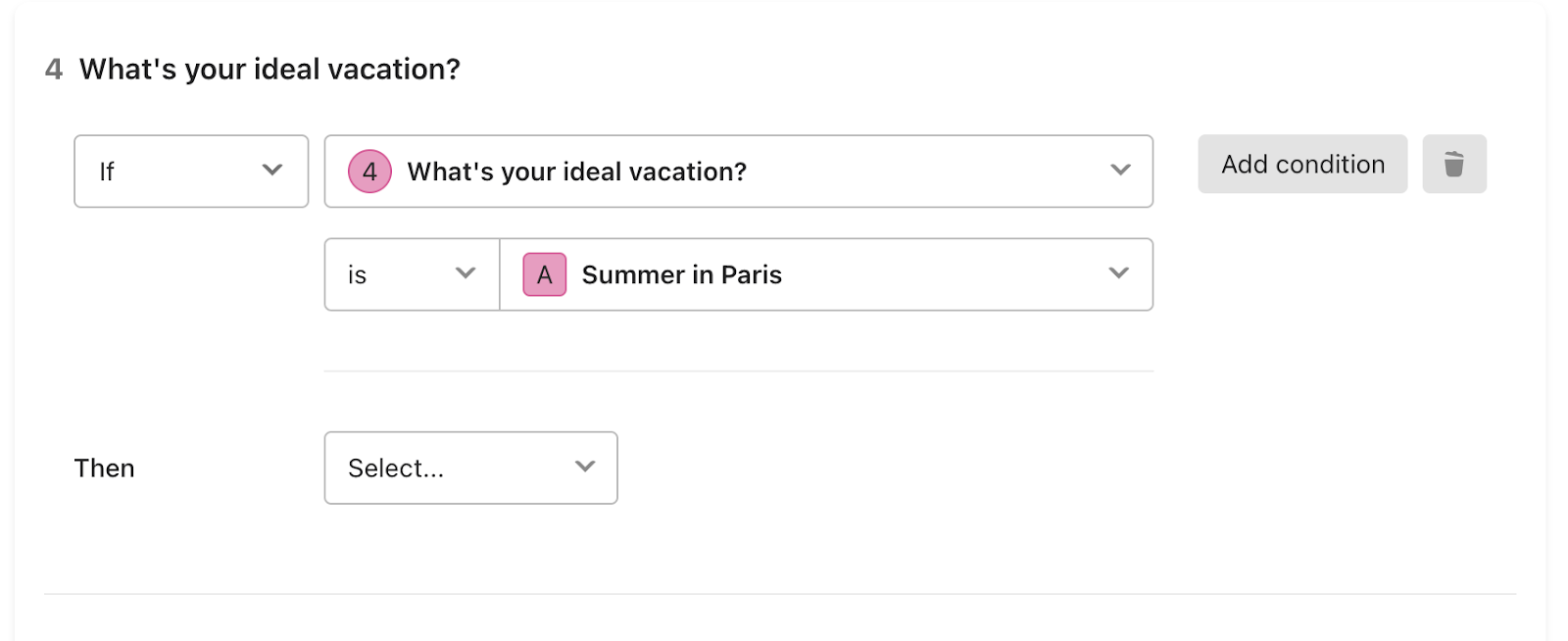Choose Replace under the second dropdown menu to change the variable’s value. You can’t do calculations on Text variables, so you won’t be able to choose a math operation.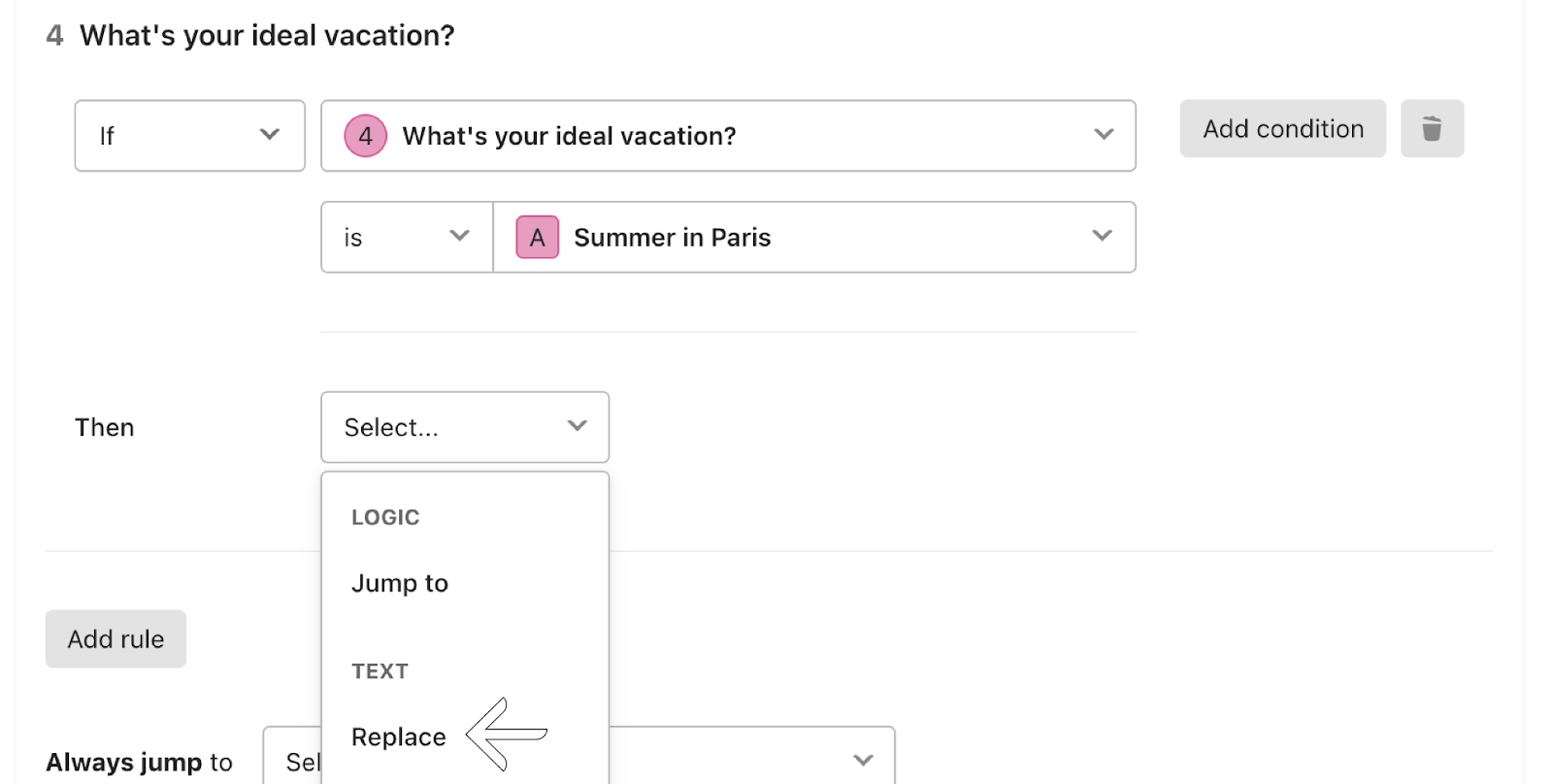Then, choose the Text variable to change and type in the new value.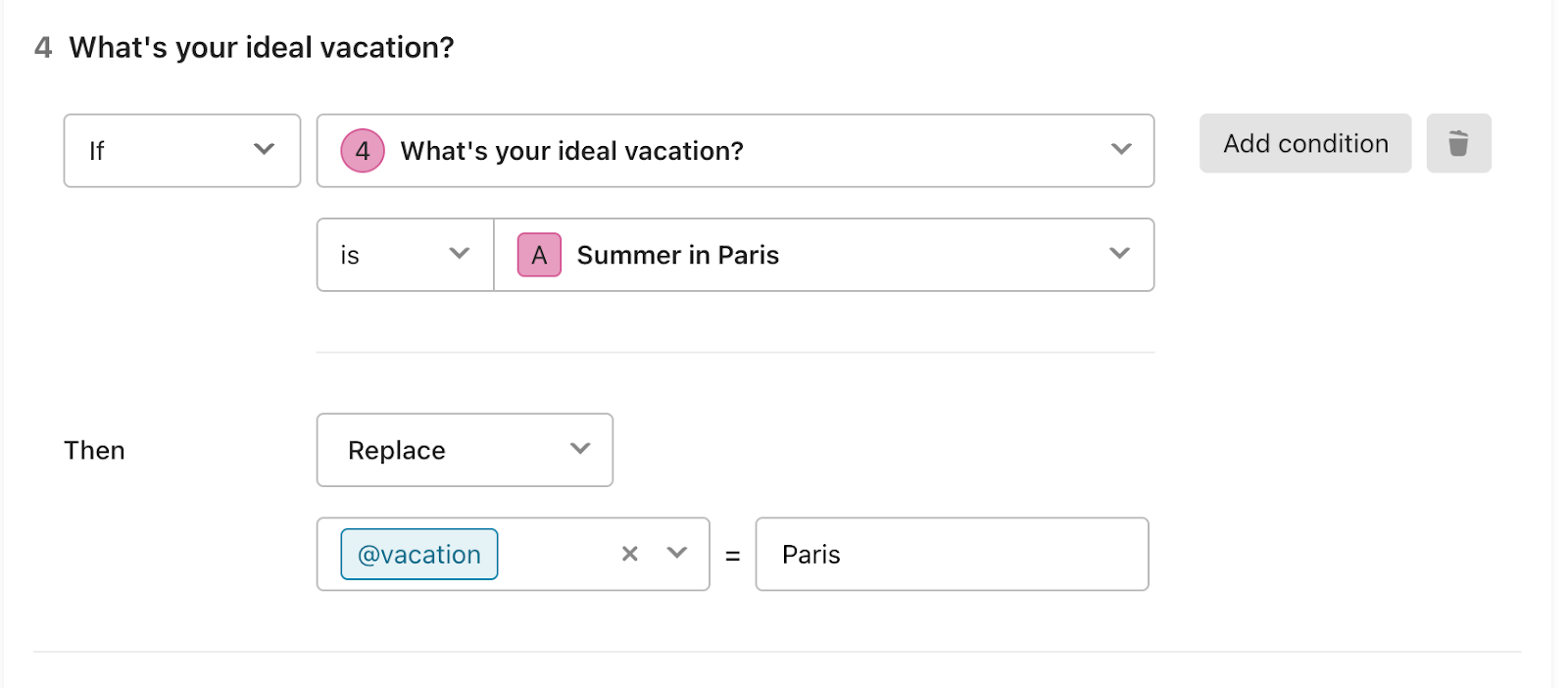######Tip: Text variables can contain alphanumeric characters (a-z, A-Z, 0-9) as well as special characters (&, !, etc.). Even if your text variable contains only numbers, it will be treated as a string of text, and you won’t be able to do calculations with it.

Want ideas for using Text variables? Check out this article on creating coupon codes with Text variables.